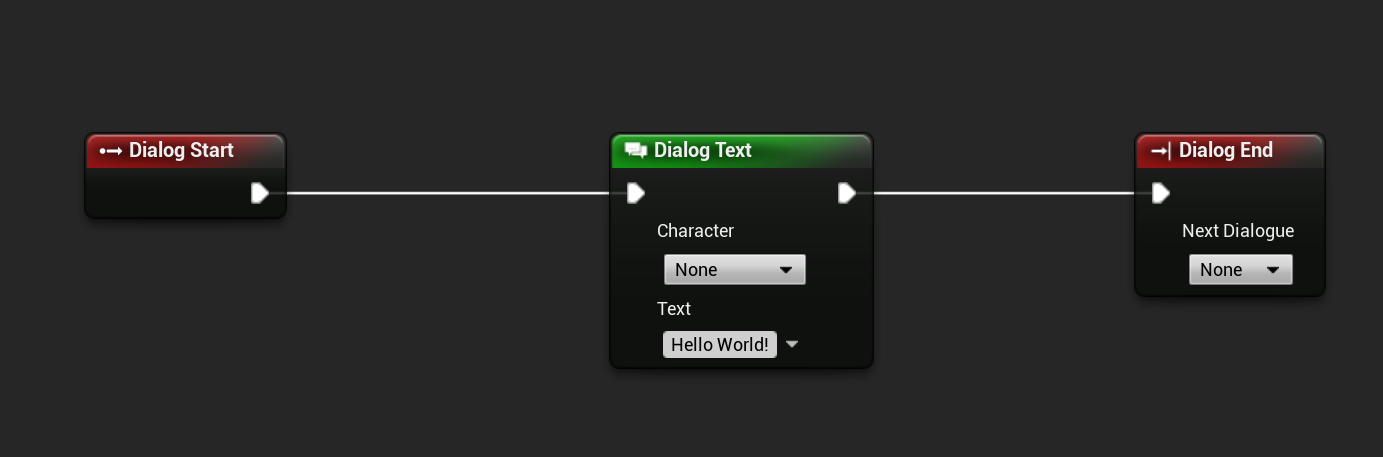# VNM Script

If the node-based approach is nothing for you, then you can also use the scripting language of Visual Novel Machinery, called VNM Script

## Basics

To use VNMScript you need to create a file called “<name>.vnm”. It is vital that the file ends if “.vnm”. If you don’t do this then the Unreal Engine will fail to import the file.

In the file you can copy the snippet from below:

``````dialog.text "Hello World!"
dialog.end
``````

This will generate the following node-based dialog in Unreal Engine:To import the VNMScript file drag and drop it into your Content Browser. After importing, don’t forget to add the new dialog to the Dialog Definitions Data Table, so that you can use it.

For reference how to use all features in VNMScript check their respective documentation page. They always contain the example as both a Blueprint Image and in form of VNMScript.

## Variables

VNMScript supports definition and usage of variables. There are multiple variable types supported in VNMScript:

• int (Integer (42))
• bool (Boolean variable (true/false))
• float (Decimal number (42.42))
• String (Text (“Text Example”))

To create them you need to use the var keyword as seen below:

``````var bool booltest
var int inttest
var string stringtest
var float floattest
``````

Integer and decimal variables support the use of arthimetic operators for performing calculations. You can see their usage in the example below:

``````var bool booltest
var int inttest
var string stringtest
var float floattest
var float floattest2

stringtest = "Test"
booltest = true
inttest = 3
inttest = 3 + 4
inttest = 3 - 4
inttest = 3 * 4
inttest = 3 / 4
floattest = 3.4
floattest = 3.4 + 2.4
floattest = 3.4 - 2.4
floattest = 3.4 * 2.4
floattest = 3.4 / 2.4
floattest2 = floattest + 2.4
``````

As seen above you can add numbers directly or use sum up another variable with a number.

Boolean variables also support logical operators like && and ||, as shown in the example below:

``````var bool booltest
var bool booltest2
var bool booltest3

booltest = true
booltest2 = false
booltest3 = booltest || booltest2
booltest3 = booltest && booltest2
booltest3 = 3 > 4
booltest3 = 3 >= 4
booltest3 = 3 < 4
booltest3 = 3 <= 4
booltest3 = 3 == 4
``````

The script parser then will import these operations and generate a Math Expression Node in the node-based dialog blueprint.

## Important Notes

If you are using Ids in your script, make sure you have added them to the respective data table before you import the file.

For example:

``````dialog.text -character=cube "Hello World as Cube!"
dialog.end
``````

Make sure you have added a character with the id cube to the Character Definitions Data Table.